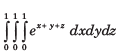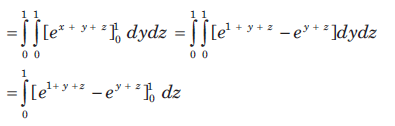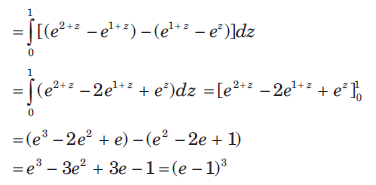Courses

# Integral Calculus - MCQ Test

## 20 Questions MCQ Test RRB JE for Electrical Engineering | Integral Calculus - MCQ Test

Description
This mock test of Integral Calculus - MCQ Test for GATE helps you for every GATE entrance exam. This contains 20 Multiple Choice Questions for GATE Integral Calculus - MCQ Test (mcq) to study with solutions a complete question bank. The solved questions answers in this Integral Calculus - MCQ Test quiz give you a good mix of easy questions and tough questions. GATE students definitely take this Integral Calculus - MCQ Test exercise for a better result in the exam. You can find other Integral Calculus - MCQ Test extra questions, long questions & short questions for GATE on EduRev as well by searching above.
QUESTION: 1

###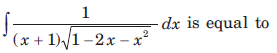​ ​ ​

Solution: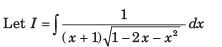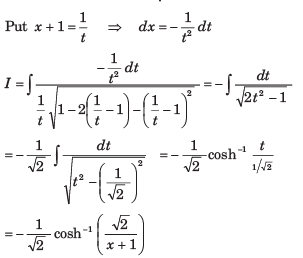QUESTION: 2

###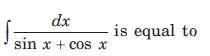​ ​ ​

Solution: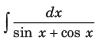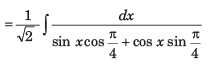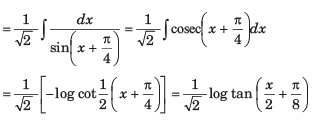QUESTION: 3

###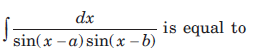Solution: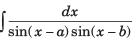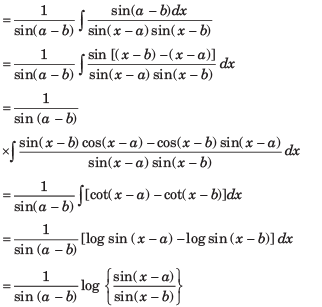QUESTION: 4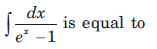Solution: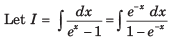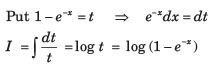QUESTION: 5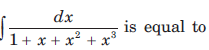Solution: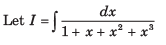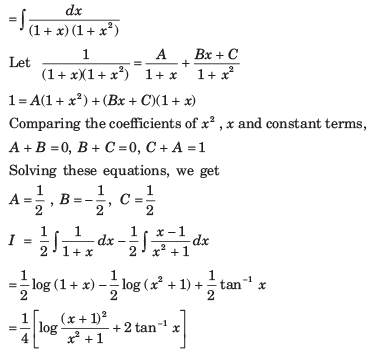QUESTION: 6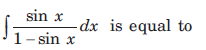Solution: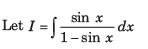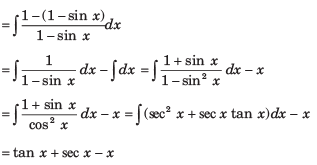QUESTION: 7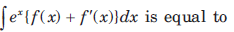Solution: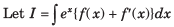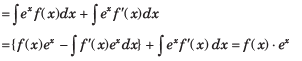QUESTION: 8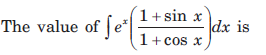Solution: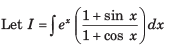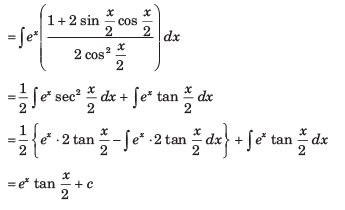QUESTION: 9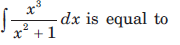Solution: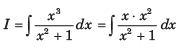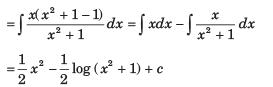QUESTION: 10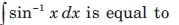Solution: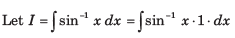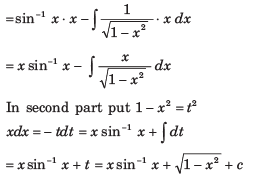QUESTION: 11

If A is the region bounded by the parabolas y2 = 4x and x2 = 4y then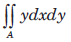is equal to

Solution: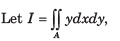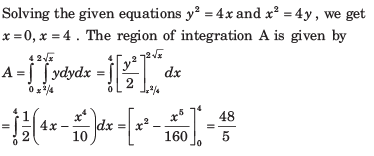QUESTION: 12

The area of the region bounded by the curves x2 + y2 = a2  and x + y = a in the first quadrant is given by

Solution:

The curves are

x2 + y2 = a2 ...(i)

and x + y = a ...(ii)

The curves (i) and (ii) intersect at A (a, 0) and B (0,a)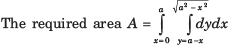QUESTION: 13

The area bounded by the curves y = 2√x , y = -x , x = 1  and x = 4 is given by

Solution:

The given equations of the curves are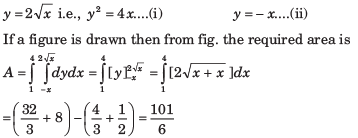QUESTION: 14

The area bounded by the curves y2 = 9x , x - y + 2 = 0 is given by

Solution:

The equations of the given curves are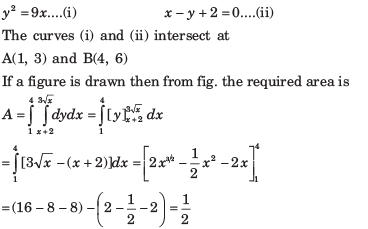QUESTION: 15

The area of the cardioid r = a (1 + cos θ) is given by

Solution:

The equation of the cardioid is

r = a (1 + cos θ)  .... (i)

If a figure is drawn then from fig. the required area is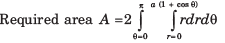QUESTION: 16

The area bounded by the curve r = θ cosθ and the lines θ = 0 and θ = π/2 is given by

Solution:

The equation of the given curve is

r = θ cosθ ...(i)

The required area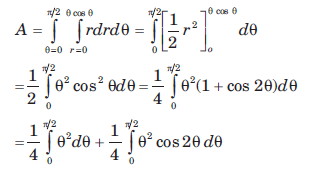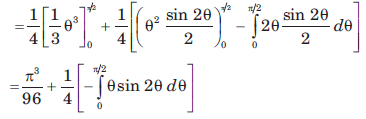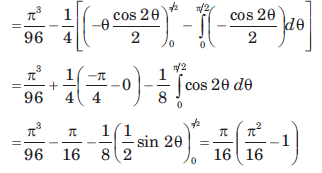QUESTION: 17

The area of the lemniscate r2 = a2 cos2θ is given by

Solution:

If a figure is drawn then from fig. the required area is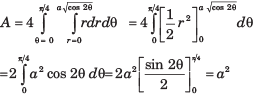QUESTION: 18

The area of the region bounded by the curve y(x2 + 2) = 3x and 4y = x2 is given by

Solution:

The equations of given curves are

y(x2 + 2) = 3x ....(i) and 4y = x2 ....(ii)

The curve (i) and (ii) intersect at A (2, 1).
If a figure is drawn then from fig. the required area is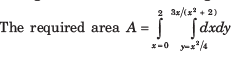QUESTION: 19

The volume of the cylinder x2 + y2 = a2 bounded below by z = 0 and bounded above by z = h is given by

Solution:

The equation of the cylinder is  x2 + y2 = a2

The equation of surface CDE is z = h

If a figure is drawn then from fig. the required area is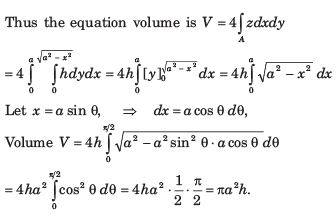QUESTION: 20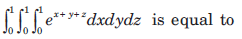Solution: